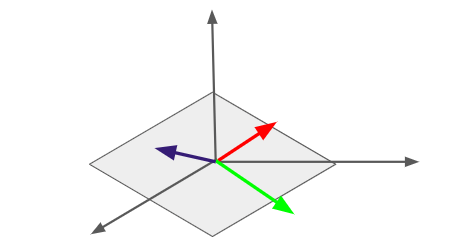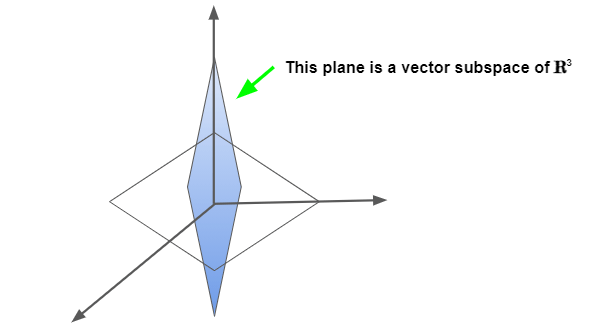As we already learnt what a linear combination is, in the previous topic, we can easily define linear independence.

Linear Independence

Definition: A list of vectors is said to be linearly independent if none of the vectors in the list can be written as a linear combination of the others.

In other words, a list of vectors is said to be linearly independent if and only if  there is no vector in the list which is in the span of the preceding vectors.

Theorem: A list of vectors is said to be linearly independent if and only if  there is no vector in the list which is in the span of the preceding vectors.

Proof:

According to the definition of Linear Independence, if a list of vectors is linearly independent, no other vector in the list can be represented as the linear combination of others. This means, no vector can be in the span of the rest of the vectors in the list.

Let’s suppose there exists a list of vectors v1 , v2 ,…, vn in which no vector is in the span of the remaining vectors. Note that such a list necessarily does not contain 0.

Assumption: The list of vectors v1, v2,…, vn is linearly dependent.

According to our assumption, one of the vectors could be written as the linear combination of the others. Without the loss of generality, let us suppose v1 is such a vector which can be written as the linear combination of the others, then:$v_1=c_2v_2+c_3v_3+.....+c_nv_n$

for some c2, c3,….,cn which are not all zero. Let ck be the last non-zero c, then we obtain:$v_k=\frac{v_1-(c_2v_2+c_3v_3+...+c_{k-1}v_{k-1})}{c_k}$

Hence, we arrive at a contradiction. Therefore, the vector-list v1, v2,…, vn must be linearly independent.

Now that we understand what linear dependence and linear independence is, let us view some geometrical examples illustrated in 3D space.

• If any two of the vectors are parallel, then it means one vector is a scalar multiple of the other. A vector being able to be written as a scalar multiple of the other is nothing but the linear combination, so the vectors are linearly dependent as demonstrated below.• If no two of the vectors are parallel among the three but all three lie in a plane, then any two of those vectors will be able to span that plane. This means, the third vector is a linear combination of the first two, since it also lies in this plane, so these kinds of vectors are also linearly dependent.• If the three vectors don’t all lie in some plane at once through the origin, none is in the span of the other two, so none of the vectors is a linear combination of the other two. Such three vectors are linearly independent.Test for Linear Dependence and Independence of Vectors

We perform the following test to know if one of the vectors is some combination of the other vectors in a set.

Two vectors u and v are linearly independent if the only real numbers x and y satisfying the equation$xu+yv = 0$ are$x = y = 0$

Let’s say,$u = \begin{bmatrix}a\\b\end{bmatrix}$ and$v = \begin{bmatrix}c\\d\end{bmatrix}$, then$xu+yv = 0$ will be equivalent to$0 = x\begin{bmatrix}a\\b\end{bmatrix}+y\begin{bmatrix}c\\d\end{bmatrix} = \begin{bmatrix}a & c \\b & d\end{bmatrix}\begin{bmatrix}x\\y\end{bmatrix}$

If u and v are linearly independent, then the only solution to this system of equations is the trivial solution,$x = y = 0$. For homogeneous systems this happens precisely when the determinant is non-zero.

So, to determine whether a given set of vectors is linearly independent we perform the following test: A set of n vectors of length n is linearly independent if the matrix with these vectors as columns has a non-zero determinant while it’s dependent if the determinant is zero.

Q: Find out whether the vectors$x = [1, 2, 3], y = [3, 2, 1]$ and$z = [0, 4, 8]$ are linearly dependent or independent.

				
%use s2
// define vectors x,y,z
var x = DenseVector(arrayOf(1.0, 2.0, 3.0))
var y = DenseVector(arrayOf(3.0, 2.0, 1.0))
var z = DenseVector(arrayOf(0.0, 4.0, 8.0))

// Create a 3x3 matrix for x, y, z
val A = DenseMatrix(arrayOf(
doubleArrayOf(1.0, 3.0, 0.0),
doubleArrayOf(2.0, 2.0, 4.0),
doubleArrayOf(3.0, 1.0, 8.0)))

//find the determinant
val det: Double = MatrixMeasure.det(A)

//if det is zero, printing "LINEARLY DEPENDENT"
if (det==0.0)
println("LINEARLY DEPENDENT")
else //else printing "LINEARLY INDEPENDENT"
println("LINEARLY INDEPENDENT")


Output: LINEARLY DEPENDENT

Spans of lists of vectors are so crucial that we give them a special name: A vector space is a nonempty set
of vectors which is closed under the vector space operations. If V and W are vector spaces and V ⊂ W, then
V is called a subspace of W.

• Lines and planes through the origin are vector subspaces of$\mathbb{R}^3$. More generally, the span of any list of vectors in$\mathbb{R}^n$ is a vector subspace of$\mathbb{R}^n$.• A spanning list of a vector space V is a list of vectors in V whose span is equal to V.

Example:

The list$\left\{\begin{bmatrix}2\\1\end{bmatrix}, \begin{bmatrix}1\\1\end{bmatrix}, \begin{bmatrix}7\\11\end{bmatrix}\right\}$ is a spanning list for$\mathbb{R}^2$ because any vector$v = \begin{bmatrix}x\\y\end{bmatrix}\epsilon \mathbb{R}^2$ can be represented as:$v = (x-y)\begin{bmatrix}2\\1\end{bmatrix}+(2y-x)\begin{bmatrix}1\\1\end{bmatrix}+0\begin{bmatrix}7\\11\end{bmatrix}$

Basis

A linearly independent spanning list for a vector space V is called a basis for V.

Ex: The list$\left\{\begin{bmatrix}1\\0\end{bmatrix}, \begin{bmatrix}0\\1\end{bmatrix}\right\}$ is a basis for$\mathbb{R}^2$.

Q: Determine whether$\left\{\begin{bmatrix}2\\1\end{bmatrix}, \begin{bmatrix}1\\1\end{bmatrix}\right\}$ is a basis for$\mathbb{R}^2$.

				
%use s2
// define vectors x,y.
var x = DenseVector(arrayOf(2.0, 1.0))
var y = DenseVector(arrayOf(1.0, 1.0))

// Create a 2x2 matrix for x, y
val A = DenseMatrix(arrayOf(
doubleArrayOf(2.0, 1.0),
doubleArrayOf(1.0, 1.0)))

//find the determinant
val det: Double = MatrixMeasure.det(A)

//if det is zero, it means the set of vectors is Linearly Dependent, so it's "NOT A BASIS"
if (det==0.0)
println("($x,$y) is Not a Basis for R2")
else //else, Linearly Independent, i.e., it's a "BASIS"
println("($x,$y) is a Basis for R2")


Output: ([2.000000, 1.000000] ,[1.000000, 1.000000] ) is a Basis for R2

Theorem: If V is a vector space, then any spanning list of V is at least as long as any linearly independent list of vectors in V.

In other words, if L1 is a linearly independent list of vectors in V and L2 is a list of vectors which spans V, then the length of L1 is less than or equal to the length of L2.

Note: All bases of a vector space V have the same length.

Linear Transformation

Let$v, w$ be real vector spaces then, a linear transformation$L$ is a function from$v$ to$w$ which satisfies$L(\alpha v+\beta w) = \alpha L(v)+\beta L(w)$.

In$\mathbb{R}^2$, reflection along the line$y = x$, defined by$L\left(\begin{bmatrix}x\\y\end{bmatrix}\right) = \begin{bmatrix}y\\x\end{bmatrix}$ is linear because,$L\left(\alpha\begin{bmatrix}x_1\\y_1\end{bmatrix}+\beta\begin{bmatrix}x_2\\y_2\end{bmatrix}\right) = \begin{bmatrix}\alpha y_1+\beta y_2\\\alpha x_1+\beta x_2\end{bmatrix}$

=$\alpha\begin{bmatrix}y_1\\x_1\end{bmatrix}+\beta\begin{bmatrix}y_2\\x_2\end{bmatrix}$

=$\alpha L\left(\begin{bmatrix}x_1\\y_1\end{bmatrix}\right)+\beta L\left(\begin{bmatrix}x_2\\y_2\end{bmatrix}\right)$

Rank of a Linear Transformation

The rank of a linear transformation from one vector space to another is the dimension of its range.

If$L\left(\begin{bmatrix}x\\y\\z\end{bmatrix}\right) = \begin{bmatrix} y+z\\y-z\\0\end{bmatrix}$, then the rank of$L$ is$2$ since its range is the$xy$-plane in$\mathbb{R}^3$.

Q: Find the rank of the linear transformation$L: \mathbb{R}^3 \rightarrow \mathbb{R}^3$ defined by the matrix$A = \begin{bmatrix}1 & 0 & -2\\0 & 1 & 1\\0 & 0 & 0\end{bmatrix}$.

				
%use s2
// Create the matrix
val A = DenseMatrix(arrayOf(
doubleArrayOf(1.0, 0.0, -2.0),
doubleArrayOf(0.0, 1.0, 1.0),
doubleArrayOf(0.0, 0.0, 0.0)))
// Compute the rank
val precision = 1e-15
val rank = MatrixMeasure.rank(A, precision)
println("rank: $rank")   rank: 2 Null Space of a Linear Transformation The null space of a linear transformation is the set of vectors which are mapped to the zero vector by the linear transformation. If$L\left(\begin{bmatrix}x\\y\\z\end{bmatrix}\right) = \begin{bmatrix} y+z\\y-z\\0\end{bmatrix}$, then the null space of$L$ is span$\left(\left\{\begin{bmatrix}1\\0\\0\end{bmatrix}\right\}\right)$ since$L(v) = 0$ implies that$v = \begin{bmatrix}x\\0\\0\end{bmatrix}$ for some$x\epsilon\mathbb{R}$. Let us illustrate the above example using s2. The matrix for the above linear Transformation will be:$A = \begin{bmatrix}0 & 1 & 1\\0 & -1 & 1\\0 & 0 & 0\end{bmatrix}$ Approach: The Null Space of$A$ is the span of all vectors$x$ such that$Ax = 0$.   %use s2 // Create a matrix val A = DenseMatrix(arrayOf( doubleArrayOf(0.0, 1.0, 1.0), doubleArrayOf(0.0, -1.0, 1.0), doubleArrayOf(0.0, 0.0, 0.0))) // Create a solver for linear system val precision = 1e-15 val solver = LinearSystemSolver(precision) // solutions for Ax = 0 val soln: LinearSystemSolver.Solution = solver.solve(A) // xList is a list of possible homogeneous solutions val xList = soln.homogeneousSoln println("Null Space of A is the span of:${xList.toTypedArray().contentToString()}")


Null Space of A is the span of: [[1.000000, -0.000000, -0.000000] ]